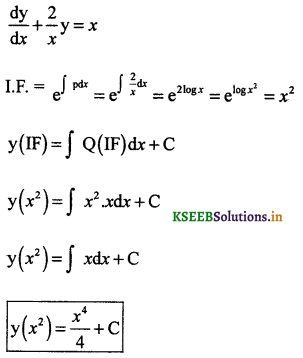# 2nd PUC Maths Question Bank Chapter 9 Differential Equations

Students can Download 2nd PUC Maths Chapter 9 Differential Equations Questions and Answers, Notes Pdf, 2nd PUC Maths Question Bank with Answers helps you to revise the complete Karnataka State Board Syllabus and to clear all their doubts, score well in final exams.

## Karnataka 2nd PUC Maths Question Bank Chapter 9 Differential Equations

### 2nd PUC Maths Differential Equations Two Marks Questions and Answers

Find the order and degree of the following differential equations.

Question 1.
y’ + 5y = 0.
The highest order derivative which occurs in the given differential equation is y’ and index of highest power is one, therefore the given differential equation is of order 1 and degree 1.

Question 2.
$$\left(\frac{d s}{d t}\right)^{4}+3 s \frac{d^{2} s}{d t^{2}}=0$$
The highest order derivative present in the given differential equation is d2s/dt2. Therefore, the index of its highest power is one, hence the given differential equation is of order 2 and degree 1.Question 3.
$$\frac{d^{2} y}{d x^{2}}$$ = cos 3x + sm3x
The highest order derivative which occurs in the given differential equation is $$\frac{d^{2} y}{d x^{2}}$$. Therefore, its order is two. it is a polynomial equation in $$\frac{d^{2} y}{d x^{2}}$$ and the power raised to $$\frac{d^{2} y}{d x^{2}}$$ is 1. Hence, its degree is one.

Question 4.
(y”’)2 + (y”)3 + (y’)4 + y5 = 0.
The highest order derivative which occurs in the given differential equation is y'”. Therefore, its order is three. The given differential equation is a polynomial equation in y'”,y” and y’. The highest power raised to y'” is 2. Hence, its degree is 2.

Question 5.
y”’+2y” + y’=0.
The highest order derivative which occurs in the given differential equation is y”’. Therefore, its order is three. It is a polynomial equation in y”’,y”and y’. The highest power raised to y”’ is 1. Hence, its degree is 1.

Question 6.
y’ + y = ex.
The highest order derivative present in the given differential equation is y’. Therefore, its order is one. The given differential equation is a polynomial equation in y’. The highest power raised to y’ is 1. Hence, its degree is 1.

Question 7.
y” + (y’)2 + 2y = 0.
The highest order derivative, present in the given differential equation is y”. Therefore, its order is two. The given differential equation is a polynomial equation in y” and y’ and the highest power raised to y” is 1. Hence its degree is 1.

Question 8.
y” + 2y’ + sin y = 0.
The highest order derivative, present in the differential equation is y”. Therefore, its order is two. This is a polynomial equation in y” and y’ and the highest power raised to y” is one. Hence, its degree is one.### 2nd PUC Maths Differential Equations Three Marks Questions and Answers

(a) Form a differential equation by eliminating arbitrary constants a and b.

Question 1.
$$\frac{x}{a}+\frac{y}{b}$$ = 1.
Given, family is $$\frac{x}{a}+\frac{y}{b}$$ = 1.
On differentiating both sides w.r.t.x. we get $$\frac{1}{a}+\frac{1}{b} \frac{d y}{d x}$$ = 0.
Again differentiating both sides with respect to x, we getwhich is the required differential equation.

Question 2.
y2 = a(b2 – x2).
Given family is y2 = a(b2 – x2)
On differentiating w.r.t.x we get
2yy’ = a (0 – 2x) ⇒ 2yy’= a (-2x) ⇒ yy’ = – ax …….(ii)
Again differentiating w.r.t.x, we get
yy” + (y’)2 = -a (using product rule of differentiation) …….(iii)
Now, for eliminating a, put the value of a from Eq. (iii) in Eq. (ii), we get
yy’ = [yy”+(y’)2]x
which is the required differeential equation.

Question 3.
y = ae3x + be-2x.
Given family is y = ae3x + be-2x …… (i)
On differentiating w. r. t.x we get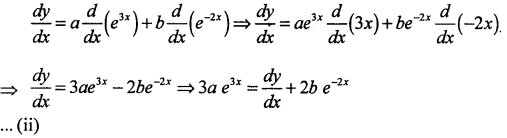Again differentiating w.r.t.x, we get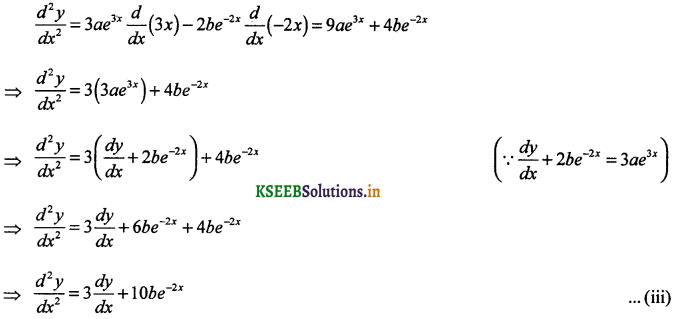On multiplying Eq. (i) by 3 and then subtrating from Eq. (ii), we getOn putting the value of b in Eq. (iii), we getwhich is the required differential equation.Question 4.
y = ex (a + bx).
Given, y = ex (a + bx) …..(i)
On differentiating both sides w.r.t.x, we get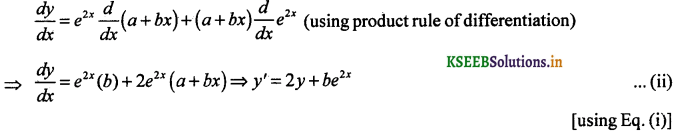Again differentiating Eq. (ii) w.r.t.x, we get
y” = 2y’ + 2be2x
On multiplying Eq. (ii) by 2 and then subtracting form Eq. (iii), we get
y” = 2y’ + 2(y’ – 2y) ⇒ y” = 2y’ + 2y’- 4y ⇒ y” – 4y’ + 4y = 0.
which is the required differential equation.

Question 5.
y = ex (a cos x + b sin x).
Given, y = ex (a cos x + b sin x). ….(i)
On dividing both sides by ex, e-xy = (a cos x + b sin x) ….(ii)
On differentiating both sides w.r.t.x, we get
e-x y1 + y (- e-x) = -a sin x + b cos x⇒ e-xy” + y’e-x(-1) – [ye-x(-1) + e-xy’] = -a cos x – b sin x
⇒ e-xy” + 2y’e-x + ye-x = -(ye-x) [using Eq. (ii)]
⇒ e-x[y”- 2y’ + 2y] = 0 ⇒ y”-2y’ + 2y = 0 [Dividing by e-x]
which is the required differential equation.

(b)

Question 1.
Form the differential equation of the family of circles touëhing the y-axis at origin.
The centre of the circle touching the y-axis at origin lies on the x-axis.
Let (a, 0) be the centre of the circle and its radius is a. Now, the equation of the family of
circule with centre (a, 0) and radius (a) is (x – a)2 + y2 = a2 ⇒ x2 + y2 = 2ax ….. (i)
On differentiating Eq. (i) w.r.x, we get 2x + 2yy’ = 2a ⇒ x + yy’ = a
Now, substituting the value of a in Eq. (i), we get
x2 + y2 = 2(x + yy’)x ⇒ x2 + y2 = 2x2 + 2xyy’ ⇒ 2xyy’ + x2 = y2
which is the required differential equation.Question 2.
Form the differential equation of the family of parabolas having vertex at origin and axis along positive y-axis.
The equation of the family of parabola having the vertex at origin and the axis along the
positive y axis is x2 = 4ay. …… (i)
On differentiating Eq. (1) w.r.t.x, we get … (ii)
On dividing Eq. (ii) by Eq. (i), we get⇒ xy’ = 2y ⇒ xy’ – 2y = 0
which is the required differential equation.

Question 3.
Form the differential equation of the family of ellipse having foci on y-axis and centre at origin.
Equation of family of ellipse is of the f0rm $$\frac{x^{2}}{b^{2}}+\frac{y^{2}}{a^{2}}=1$$ …..(i)
[Since, foci is on y-axis, so we draw a vertical ellipse]
On differentiating Eq. (i) w.r.t. x, we getAgain differentiating w.r.t x, we get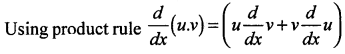⇒ x(y’)2 + xyy”- yy’ = 0 ⇒ xyy” + x(y’)2 – yy’ = 0
which is the required differential equation.

Question 4.
Form the differential equation of the family of hyperbolas having foci on x-axis and centre at origin.
The equation of the family of hyperbolas with the centre at origin and foci along the x – axis is $$\frac{x^{2}}{a^{2}}-\frac{y^{2}}{b^{2}}=1$$ …..(i)
On differentiating both sides w.r.t.x, we get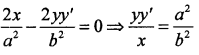Again differentiating w.r.t.x, we get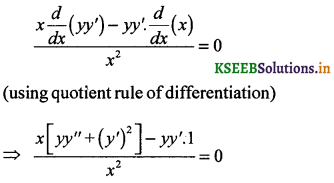(using product rule of differentiation)
⇒ x(y’)2 + xyy”- yy’ = 0 ⇒ xyy’ + x (y’)2 – yy’ = 0
which is the required differential equation.Question 5.
Form the differential equation of the family of circles having centre on y-axis and radius 3 unit.
The equation of the family circles having centre on y-axis and radius 3 unit is
x2 + (y – b)2 = 9 ….(i)
On differentiating Eq. (i) w.r.t.x, we get
2x + 2(y – b) y’= 0 ⇒ y – b = $$-\frac{x}{y^{\prime}}$$ ….(ii)
On substituting this value of (y – b) in Eq. (i) we get
x2 + $$\left(-\frac{x}{y^{\prime}}\right)^{2}$$ = 9
⇒ x2 [(y’)2 + 1] = 9 (y’)2 ⇒ (x2 – 9) (y’)2 + x2 = 0
which is the required differential equation.

Question 6.
In a bank principal in creases at therateof r% per year. Findthe value of r if ₹100 double itself in 10 yr (loge 2 = 0.6391).
Let P, t and r represent the principal, time and rate of interest respectively.
It is given that the principal increases continuously at the rate of r% per year.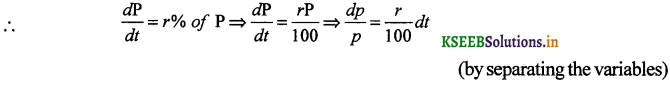On integrating both sides, we obtain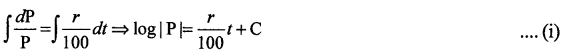When t = 0 (initialy), let P = P0, then
log |P0| = 0 + C ⇒ C = log |P0|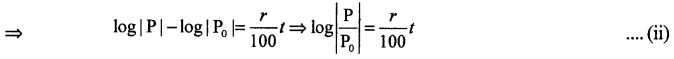It is given that when t = 10, then P = 2P0, put in Eq. (ii), we get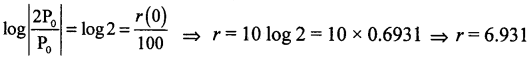Hence, the value of r is 6.93%.Question 7.
In a bank principal increases at the rate of 5% per year. An amount of ₹ 1000 is deposited with this bank, how much will it be worth after 10 yrs. (e0.5 = 1.648)?
Let P and t be the principal and time respectively.
It is given that the principal increases continuously at the rate of r% per year.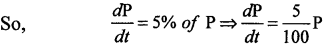On separating the varable, we get $$\frac{d \mathrm{P}}{\mathrm{P}}=\frac{1}{20} d t$$.
On integrating we get $$\int \frac{d P}{P}=\int \frac{1}{20} d t$$ ⇒ log |p| = $$\frac{1}{20}$$t + c
When t = 0, P = 1000 (initialy), this log 1000 = C
Put the value of C in Eq. (i), we get log | P |= $$\frac{1}{20}$$t + log 1000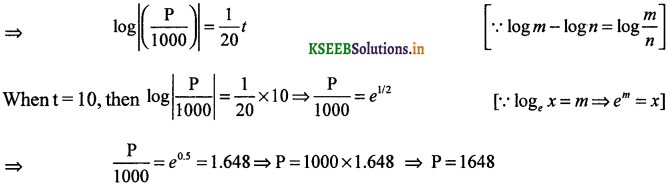Hence, after 10 yr, the amount will worth ₹1648.

### 2nd PUC Maths Differential Equations Five Marks Questions And Answers

(a) Solution of a differential equation by the method of separation of variables
Solve :

Question 1.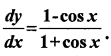⇒ y = ∫(sec2 (x/2) – 1) dx ⇒ y = ∫sec2 (x/2)dx – ∫1 dx
⇒ y = 2 tan $$\frac{x}{2}$$ – x + c.
which is the required general solution.Question 2.
$$\frac{d y}{d x}=\sqrt{4-y^{2}}$$
Given, $$\frac{d y}{d x}=\sqrt{4-y^{2}}$$
On separating the variable, we get dx = $$\frac{d y}{\sqrt{4-y^{2}}}$$
On integrating, we get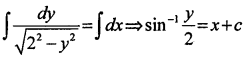⇒ $$\frac{y}{2}$$ = sin(x + c) ⇒ 2 sin (x + c) which the required general solution.

Question 3.
$$\frac{d y}{d y}$$ + y = 1(y ≠ 1)
Given $$\frac{d y}{d y}$$ + y = 1
On separatingthevariables,we get dy + ydx = dx ⇒ dy = dx – ydx
⇒ dy = dx(1 – y) ⇒ dx = $$\frac{d y}{1-y}$$
On integrating both sides, we get $$\int \frac{d y}{(1-y)}=\int d x$$
⇒ -log|1 – y| = x – C ⇒ -x + C = log|1 – y|
⇒ |1 – y| = e-x+c
⇒ y – 1 = (±ec)e-x ⇒ y= 1 + Ae-x [where, (A = ±ec)
which is the required general solution of the given differential equation.

Question 4.
sec2 x tan y dx + sec2 y tan x dy = 0.
Given sec2 x tan y dx + sec2 y tan x dy = 0
On separating the variables, we get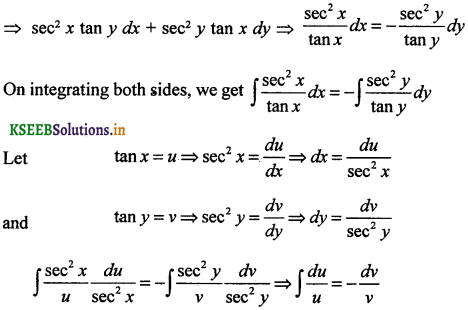⇒ log |u| = – log lv| + log |C|
⇒ log |tan x tan y| = e-x+c
⇒ log |tan x tan y| = log |C|
⇒ tan x tan C
which is the required general solution.

Question 5.
(ex + e-x)dy – (ex – e-x) dx = 0
Given (ex + e-x)dy – (ex – e-x) dx = 0
⇒ (ex + e-x)dy – (ex – e-x) dx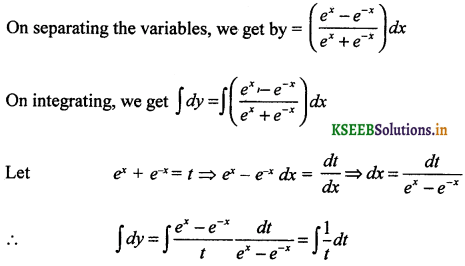⇒ y = log |t| + C ⇒ y = log|ex + e-x| + C
which is the required general solution.Question 6.
$$\frac{d y}{d x}$$ = (1 + x2)(1 + y2)
$$\frac{d y}{d x}$$ = (1 + x2)(1 + y2)
On separating the variables, we get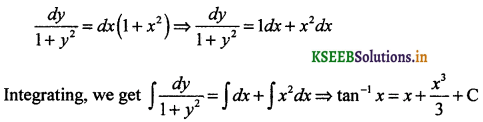which is the required general solution.

Question 7.
y log y dx – x dy = 0.
Given, y log y dx – x dy = 0
On separating the variables, we get y log y dx = x dy ⇒ $$\frac{1}{x}$$dx = $$\frac{1}{y \log y}$$ dy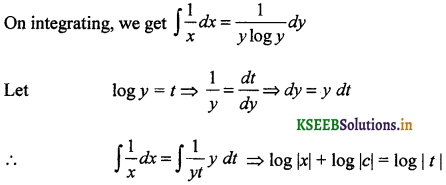⇒ log |cx| = log |log y|
⇒ cx = log y
⇒ y = ecx
which is the required general solution.

Question 8.
x5$$\frac{d y}{d x}$$ = -y5.
Given, x5$$\frac{d y}{d x}$$ = -y5
On separating the variables, we get $$\frac{d y}{y^{5}}=-\frac{d x}{x^{5}}$$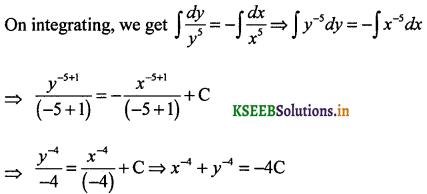which is the required general solution.Question 9.
$$\frac{d y}{d x}$$ = sin-1x
Given, $$\frac{d y}{d x}$$ = sin-1x
On separating the variables, we get dy = sin-1 x dx
On integrating, we get ∫dy = ∫sin-1 x dxwhich is the required general solution.

Question 10.
ex tan y dx + (1 – ex) sec2 y dy = 0.
ex tan y dx + (1 – ex) sec2 y dy = 0
On separating the variables, we get e* tan y dx = – (1 – ex) sec2y dy⇒ log |t| = -log |v| + log |C|
⇒ log |ex – 1| = log |tan y|- log |C|
⇒ log |C(ex – 1)| = log |tan y|
⇒ C (ex – 1) = tan y
which is the required general solution.(b) Solution of homogeneous differential equations.
Solve :

Question 1.
(x2 + xy) dy = (x2 + y2) dx.
Given, differential equation is $$\frac{d y}{d x}=\frac{x^{2}+y^{2}}{x^{2}+x y}$$ …. (i)
Here, numerator and denominator both have polynomial of degree 2. So, the given diffiential equation is homogeneous.
Let y = vx ⇒ $$\frac{d y}{d x}$$ = v + x $$\frac{d v}{d x}$$
On putting the values of $$\frac{d y}{d x}$$ and y in Eq. (i), we get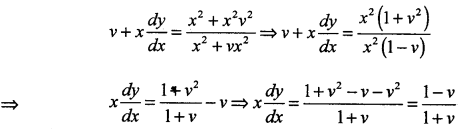On integrating both sides, we get⇒ – 2 log (1 – v) – v = log x – log C
⇒ – v – 2 log (1 – v) – log x = – logC
⇒ v + 2 log (1 – v) + log x = log CThis is the required solution of the given differential equation.Question 2.
y’ = $$\frac{x+y}{x}$$
Given y’ = $$\frac{d y}{d x}=\frac{x+y}{x}$$
Here, the given differential equation is homogeneous.
So, put y = vx ⇒ $$\frac{d y}{d x}$$ = v + x $$\frac{d v}{d x}$$
On putting values of $$\frac{d y}{d x}$$ and y in Eq. (1), we get⇒ y = x log |x| + Cx
This is the required solution of the given differential equation.

Question 3.
(x – y)dy – (x + y) dx = 0.
Given, (x – y) dy – (x + y) dx = 0
⇒ (x – y)dy = (x + y)dx ⇒$$\frac{d y}{d x}=\frac{x+y}{x-y}$$ ……(i)
Thus, the given differential equation is homogeneous,
So, put y = vx ⇒ $$\frac{d y}{d x}$$ = v + x $$\frac{d v}{d x}$$
On putting values of $$\frac{d y}{d x}$$ andy in Eq. (1), we get⇒ tan-1 v – $$\frac { 1 }{ 2 }$$ log |t|= log |x| + C
⇒ 2 tan-1 v – [log(1 + v2) + 2 log(x)] = 2C (put t = 1 + v2)
⇒ 2 tan-1v – log [(1 + v2)x2] = 2C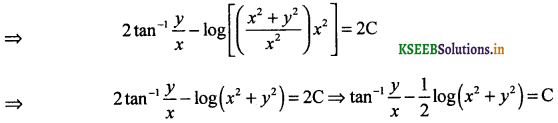This is the required solution of the given differential equation.Question 4.
(x2 – y2) dx + 2xy dy = 0.
Given, (x2 – y2) dx + 2xy dy = 0
⇒ 2xy dy = (y2 – x2) dx ⇒ $$\frac{d y}{d x}=\frac{y^{2}-x^{2}}{2 x y}$$ …..(i)
Thus, the given differential equation is homogeneous,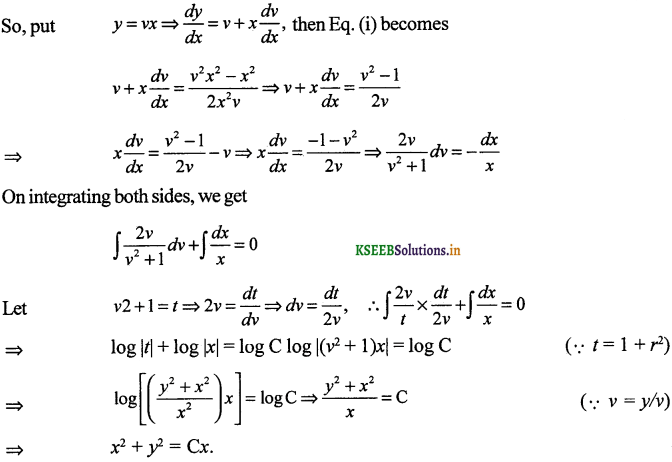This is the required solution of the given differential equation.

Question 5.
x2 $$\frac{d y}{d x}$$ = x2 – 2x2 + xy.
Given, $$\frac{d y}{d x}=\frac{x^{2}-2 x^{2}+x y}{x^{2}}$$ ….(i)
Thus, the given differential equation is homogeneous,On integrating both sides, we getThis is the required solution of the given differential equation.Question 6.
x dy – y dx = $$\sqrt{x^{2}+y^{2}}$$ dx.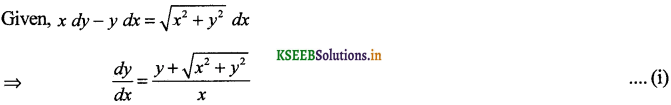Thus, the given differential equation is homogeneous,
so, put y = vxwhich is the required solution.Question 7.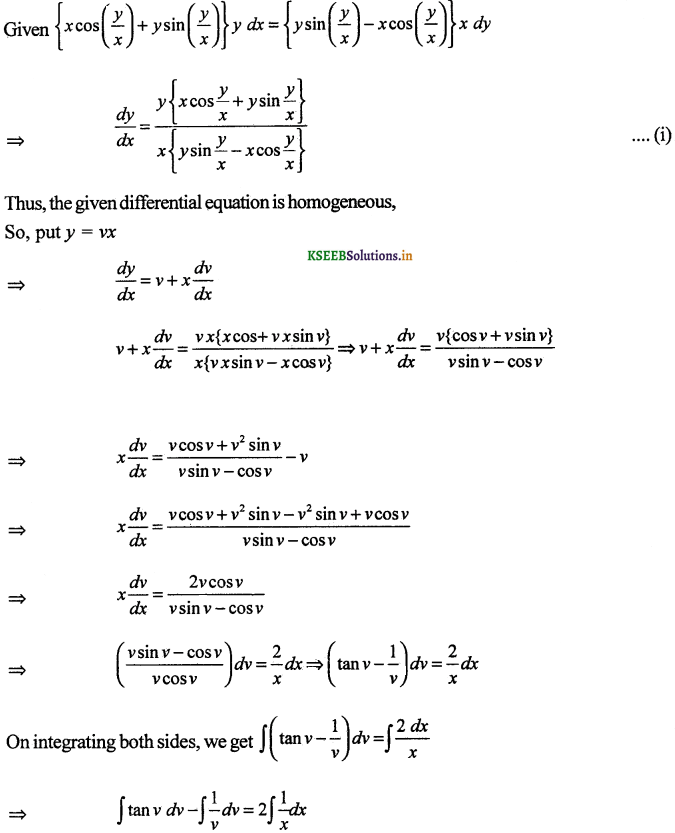⇒ log |cos v| – log |v| = log |x| + C
⇒ log |v cos v| + 2 log |x| = – C
⇒ log [(vcos v)x2] = – C ⇒ (y cos v)x2 = e-c
⇒ x2 v cos v = A (where, A = e-c)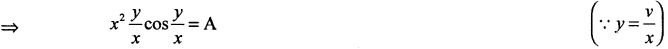⇒ xy cos $$\frac{y}{x}$$ = A
This is the required solution of the given differential equation.Question 8.Thus the given differential equation is homogeneous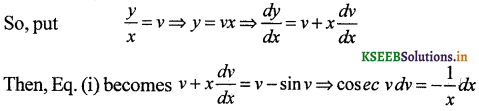On integrating both sides, we get
∫cosec v dv = -∫$$\frac{d x}{x}$$
⇒ log |cosec v – cot v| = – log |x| + A
⇒ log |(cosec v – cot v)| + log |x| = A ⇒ log |(cosec v – cot v) x| = A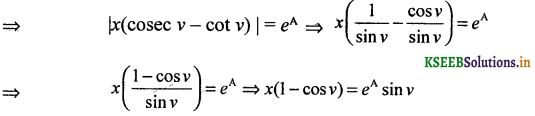This is the required solution of the given differential equation.(c) Solution of linear differential equations
Solve :

Question 1.
Solve $$\frac{d y}{d x}$$ + 3y = e-2x
Comparing with $$\frac{d y}{d x}$$ + Py = Q we get P = 3 , Q = e-2xSolution is
y(IF) = ∫Q(lF)dx + C
ye3x = ∫e-2xe3x dx + C
ye3x = ∫ex dx + C
ye3x = ex + C
y = e-2x + cee-3x

Question 2.
Solve $$\frac{d y}{d x}+\frac{y}{x}$$ = x2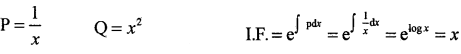y(IF) = ∫Q(lF)dx + C
yx = ∫x2x dx + C
xy = ∫x3 dx + C
xy = $$\frac{x^{4}}{4}$$ + C

Question 3.
$$\frac{d y}{d x}$$ + sec xy = tan x (0 ≤ x < $$\frac{\pi}{2}$$)
P = sec x
Q = tanx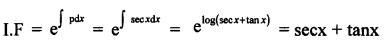y(IF) = ∫Q(lF)dx + C
y (sec x + tanx) = ∫tan x(sec x + tan x)dx
= ∫sec x tan x dx + ∫tan2xdx
= sec x + ∫(sec2x – 1)dx
= sec x + tan x – x + C
∴ y(sec x + tan x) = sec x + tan x-x + CQuestion 4.
x$$\frac{d y}{d x}$$ + 2y = x2 log x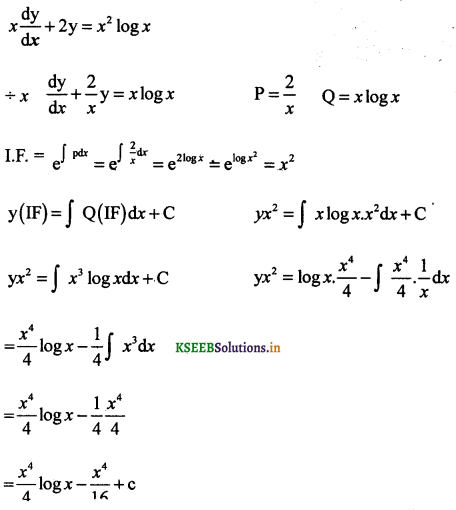Question 5.
x log x $$\frac{d y}{d x}$$ + y = $$\frac{2}{x}$$ log xQuestion 6.
(1 + x2)dy + 2xydx = cot xdxy(1 + x2) = ∫cot xdx + C
y(1 + x2) = log(sinx) + C

Question 7.
(x + y)$$\frac{d y}{d x}$$ = 1Question 8.
y dx + (x – y2)dy = 0Question 9.
(x + 3y2)$$\frac{d y}{d x}$$ = y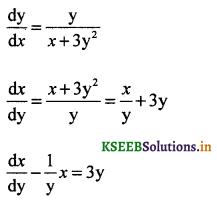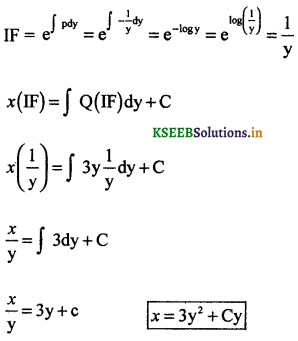x $$\frac{d y}{d x}$$ + 2y = x (x ≠ 0)